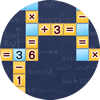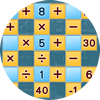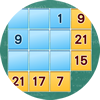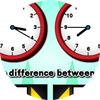Novel Games - Mastering All the Games in Human History
search Play Games Forums License Our Games
Name
0 / 2645
0
99

Settings Log Out

English

Math Crossword

## Game Description

This game is like a crossword puzzle game, but instead of words there are mathematical equations, and instead of letters there are numbers and mathematical signs. There will be some numbers and signs given, and you need to drag those to the blank spaces so that all the horizontal and vertical lines are correct mathematical equations. Note that a valid equation is not only of the form 1 + 2 = 3, but a form like 2 + 2 = 1 + 3 is also valid, but there must not be more than one equal sign in each equation. Another tricky part is that you can form a double digit number by putting two numbers together, sometimes you need to think about multiple digit numbers in order to solve the puzzle. There are multiple levels and there will be more equations in later levels. If you cannot solve a level within a time limit, then the game is over. The higher your level and the faster you solve them, the higher your rank.

Submit
Submit comment failed, please try again Submit
None Yet(Translated by #[PERSON])
(Original)
by %s
Like

None Yet
by %s

## You may also like:Garam
Put numbers into the rings to make the formulas correct.
Version: 1.0.0Math Square
Put in numbers to make the formulas correct.
Version: 1.0.0FubukiTime Difference